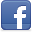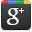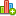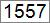Hello LearnerContributor
Stats
 Views : 73177 Comments : 0 Rating : 0
Actions

# If a five digit number is input through the keyboard.Write a .NET program to calculate the sum of its digits (hint: use the modulus operator)

By Ashok Nalam on 24 Jun 2013 | Category: C# | Tagged: .NET number
Solve the problem in the programming language .NET. If a five digit number is input through the keyboard.Write a program to calculate the sum of its digits (hint: use the modulus operator)Add Comment   |Rate this resource

Today I found one of programming question in the forums that "Solve the problem in the programming language .NET. If a five digit number is input through the keyboard.Write a program to calculate the sum of its digits (hint: use the modulus operator)". So we will see how to sum the 5 digit number using modulus operator(%).

Input is 12345 then the output should be 1+2+3+4+5=15.

#### Program:

```    class SumOf5DigitsProgram
{
public static void Main()
{
int sum = 0;
int number = 12345;
int tempnumber = number;
while (tempnumber > 0)
{
sum += (tempnumber % 10); // adds last digit of tempnumber to sum
tempnumber /= 10; // divides tempnumber by 10 to cuts the last digit
}
Console.Write("Sum of {0} digit is {1}",number,sum);
}
}```

#### Code Execution Steps:

number : 12345
tempnumber  = 12345
sum = 0

Iteration1 : 12345>0 - True
sum  = 0 + (12345%10) = 0 + 5 = 5
tempnumber = 12345/10 = 1234

Iteration2: 1234>0 - True
sum  = 5 + (1234%10) = 5 + 4 = 9
tempnumber = 1234/10 = 123

Iteration3: 123>0 - True
sum  = 9 + (123%10) = 9 + 3 = 12
tempnumber = 123/10 = 12

Iterration3: 12>0 - True
sum  = 12 + (12%10) = 12 + 2 = 14
tempnumber = 12/10 = 1

Iteration4: 1>0 - True
sum  = 14 + (1%10) = 14 + 1 = 15
tempnumber = 1/10 = 0

Iteration5: 0>0 - False
sum = 15

#### Output:

Sum of 12345 digit is 15Add CommentRate this resource
 Name Email WebSite